Get instant live expert help with Excel or Google Sheets“My Excelchat expert helped me in less than 20 minutes, saving me what would have been 5 hours of work!”

#### Post your problem and you’ll get expert help in seconds.

Your message must be at least 40 characters
Our professional experts are available now. Your privacy is guaranteed.

# How to Calculate the Next Anniversary Date in ExcelRead time: 20 minutes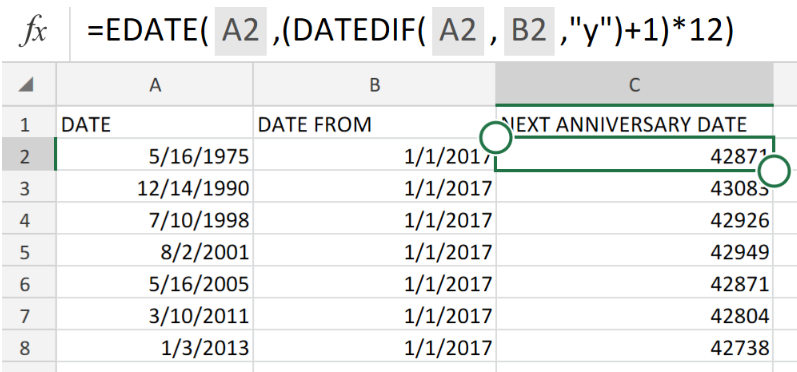Figure 1. Next Anniversary Date in Excel.

We are going to make use of a combination of the Excel EDATE and DATEDIF functions in order to determine the next anniversary date between a specified start and end date in days, months, or years.

## Generic Formula

`=EDATE(date,(DATEDIF(date,as_of,"y")+1)*12)`

• date is the specified date from which Excel begins to count.
• as_of is the specified date when Excel stops counting (after the anniversary).

The letter ”y” in our modified formula above is for specifying the difference in years.

## How to use the Excel EDATE and DATEDIFF Functions

We are now going to demonstrate how we can use the Excel EDATE and DATEDIFF Functions to get the anniversary date starting from a specific date.

This is possible in 3 simple steps.

1. Label the columns of our worksheet and enter the date specified start and end dates accordingly.

See example illustrated below;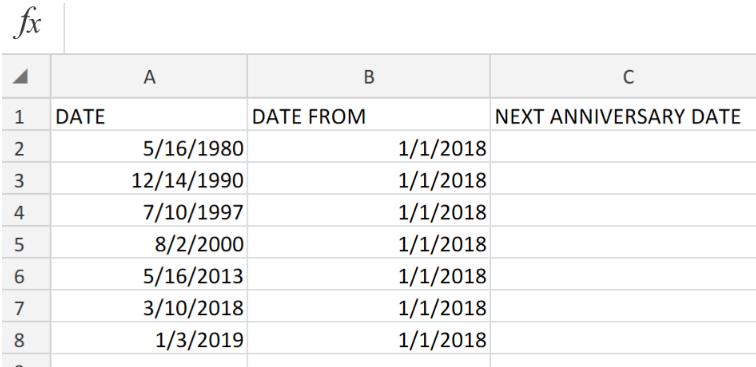Figure 2. Next Anniversary Date in Excel.

Ensure that we have blank cells in our NEXT ANNIVERSARY DATE column for Excel to return the calculated dates.

1. In our example above, the formula to enter into cell C2 is shown below;

`=EDATE(A2,(DATEDIF(A2,B2,"y")+1)*12)`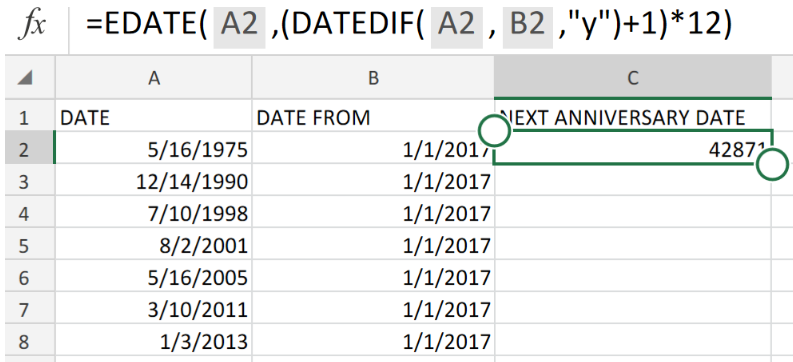Figure 3. Next Anniversary Date in Excel.

Excel DATEDIF function will determine the total number of years occurring between the start date (original anniversary date) and the end date (“as of” date),:

In our worksheet example, we have specified 1/1/2017 as our end (as of) date.

1. Copy the modified formula in cell C2 down into the remaining cells in our NEXT ANNIVERSARY DATE column to achieve the desired results.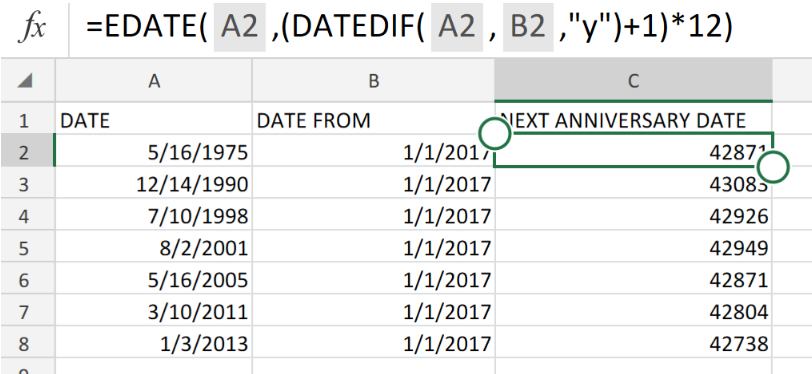Figure 4. Next Anniversary Date in Excel.

## Note

• We have used the letter “y” in our formula for Excel to calculate the difference in years.
• For Excel to calculate the difference in months, we should use the letter “m”.
• For Excel to calculate the difference in days, we should use the letter “d”.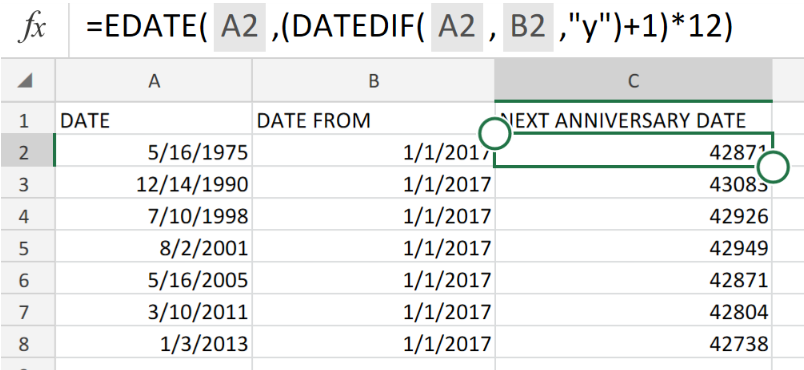Figure 5. Final Result.

## Instant Connection to an Expert through our Excelchat Service:

Our live Excelchat Service is here for you. We have Excel Experts available 24/7 to answer any Excel questions you may have. Guaranteed connection within 30 seconds and a customized solution for you within 20 minutes

### Did this post not answer your question? Get a solution from connecting with the expert.Another blog reader asked this question today on Excelchat:
Solution examplesI have multiple columns on my spread sheet and want to switch from A-Z to Z-A based on values in column A
Solved by V. H. in 38 minsConcat formula is incorrectly formatting the date in column C. Currently column B has dd/mm/yyyy and I require mm/dd/yyyy
Solved by Z. Y. in 31 minsI need a datedif function that calculates the difference in 2 dates and if it returns more than 5 years, I need it to say yes and if not no.
Solved by O. Y. in 14 minsI need a formula that will allow me to calc before a certain date and after a certain date. =if(A2<=DATE(2015,8,27),(F2*1.14),(F2*1.15)) It just multiplies column F with 1.15. I'm assuming I need to bring in the date column into the equation?
Solved by M. Q. in 20 minsI need assistance setting up a time sheet formula where I can create a column of contiguous dates down the left hand side of the spread sheet. So I can copy a contiguous column of dates, rather than entering each date manually. I would only need to have work days in the list, ie Monday to Friday inc. with a couple of spare lines between each week.
Solved by Z. Y. in 40 mins## Subscribe to Excelchat.coAnother blog reader asked this question today on Excelchat: